This service is intended for detection of total (i.e. how many goals will be scored) in the upcoming match, using the latest teams’ games. The whole service is divided into two forecasts - Step1 and Step2.

The First forecast (Step1) displays the average goal scoring level of rival teams.

The level can be from -10 (the lowest level) to +10 (the highest level). So, the closer the number approaches 10, the bigger the probability of scoring more goals. And the closer the level approaches -10, the more likely, the teams will not score.

As a basis (according to statistical indicators) there were adopted the following rules:

1) If the forecast (Step1) for the match is from 5 to 10, the recommended bet for the total is more than 2.5, i.e. there will be scored 3 or more goals.

2) If the forecast (Step1) for the match is from -5 to -10, the recommended bet for the total less than 2.5, i.e. there will be scored less than 3 goals.

As an example, there are several match day results, where the first forecast (Step1) showed a value from 5 to 10.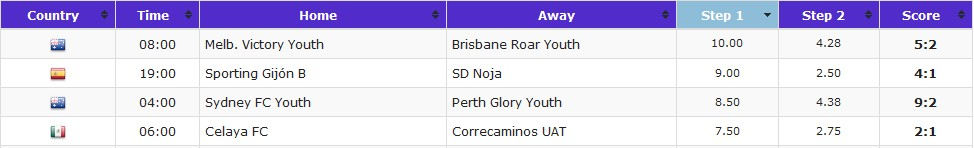The following example shows the results of the matches on the same day: the first forecast (Step1) showed values from -5 to -10.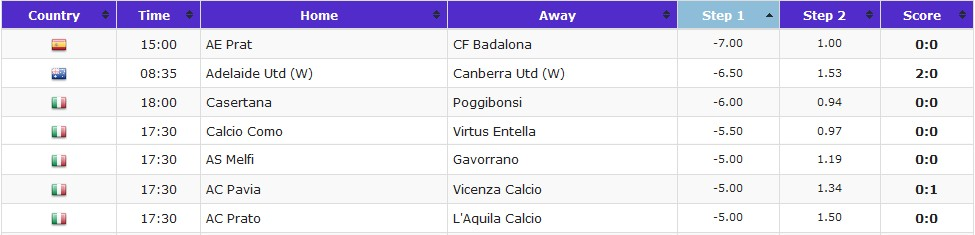As to the intermediate values ​​of the level for the game, there are all values ​​in the range from -4.99 to 4.99. They will be also useful for trends determination of the teams’ form from game to game. They can be used as a secondary, subsidiary forecast for the determination of the match’s total.

Second forecast (Step2) determines the average value of goals in the upcoming match by a simple mathematical calculation, based on the average scores of both teams’ last games.

Simply speaking, the Step2 shows the average number (in goals) for the match. This value takes on the value from 0 to the average total of both teams.

As a basis (according to statistical indicators) there were adopted the following rules:

If the forecast (Step2) for the match is from 2.5 and more, the recommended bet for the total is more than 2.5, i.e. there will be scored 3 or more goals in the match.

If the forecast (Step2) for the match is 1.5 and less, the recommended bet for the total is less than 2.5, i.e. there will be scored less than 3 goals in the match.

Let's look at the examples and consider the match day results, based only on Step2, which values ​​are less than 1.5.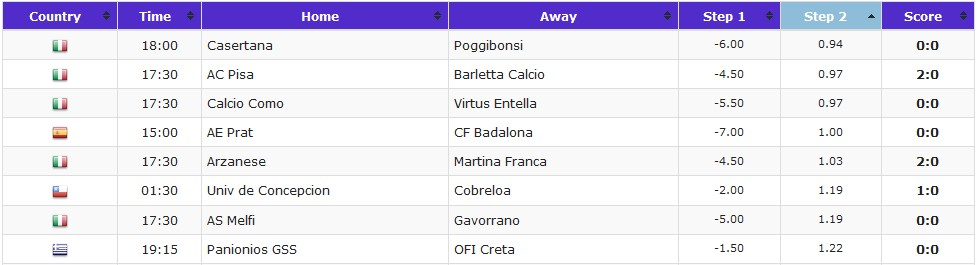And there is an example of matches in which the value of Step2 is from 2.5 and more.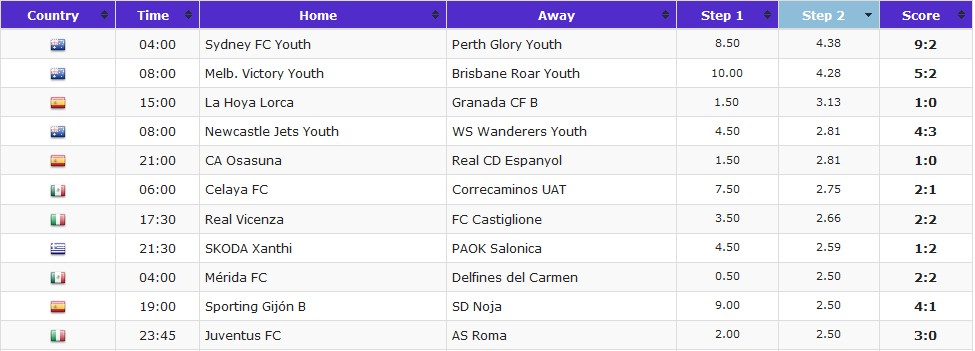As you can see, the forecasts not always give the right result.

Therefore for increasing the probability of total’s right results:

! Use Step1 and Step2 together that both forecasts recommend the same total for the match.

Let's look at the matches from the previous example, in which the value of Step2 suite to the total more than 2.5, but Step1 is not always suitable: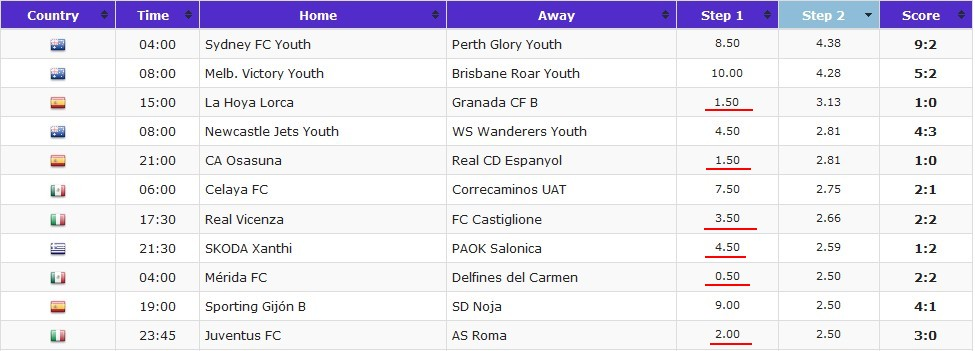Matches, which answer to the recommendations of Step2, are more than 2.5 on total, but they are not suitable to Step1. They are underlined in red.

As you can see, if we didn’t take them to this criterion, we wouldn’t have any loss this day. So, it is better to secure yourself and choose the match in which both forecasts recommend the same than to ignore one of them – seeking for quantity, but not for quality.

Of course, it happens that both forecasts show a recommendation for one result, and eventually it happens to the contrary - but it is sport. And any service cannot guarantee you 100% success in your enterprise.

Regardless of the rules, using this service with analytics of other services, it will be reached a positive result.